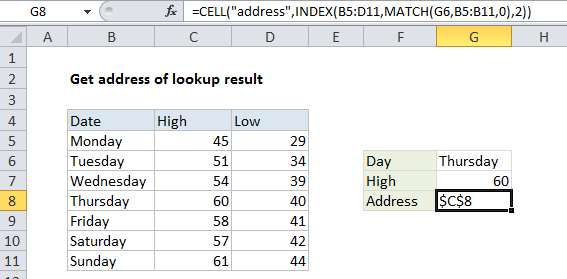## Excel Office

Excel How Tos, Tutorials, Tips & Tricks, Shortcuts

# Get address of lookup result in Excel

This tutorial shows how to Get address of lookup result in Excel using the example below;

## Formula

`=CELL("address",INDEX(range,row,col))`## Explanation

To get the address of a lookup result derived with INDEX, you can use the CELL function.

In the example shown, the formula in cell G8 is:

`=CELL("address",INDEX(B5:D11,MATCH(G6,B5:B11,0),2))`

Which returns an address of \$C\$8, the address of the cell returned by INDEX.

Also See:   INDEX and MATCH descending order in Excel

### How this formula works

Although INDEX normally displays the value of a cell at a given index, underneath it actually returns a reference.

By wrapping INDEX in the ADDRESS function, you can see the address of the cell returned by the lookup.